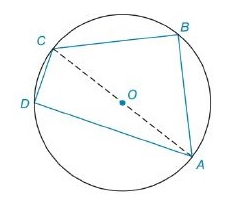Chapter 8.2, Problem 27E### Elementary Geometry for College St...

6th Edition
Daniel C. Alexander + 1 other
ISBN: 9781285195698

#### Solutions

Chapter
Section### Elementary Geometry for College St...

6th Edition
Daniel C. Alexander + 1 other
ISBN: 9781285195698
Textbook Problem
1 views

# In Exercises 27 to 30, find the area of the figure shown. Given: In ⊙ O , O A = 5 , B C = 6 , a n d C D = 4 Find: A A B C DTo determine

To Find:

The area of the quadrilateral ABCD inscribed by the circle with centre 'O'.

Explanation

Formula Used:

Angle subtended by a diameter on any point of circle is 90°.

Pythagorean theorem for the right angle triangle ABC for the hypotenuse AC,

AC2=AB2+BC2.

Area of right triangle =12×b×h

Calculation:

This quadrilateral can be divided into two triangles. That is, ABC and ADC.

By the angle subtended in a diameter of the circle theorem, it makes 90° at the mD and mB.

It is given that OA=5=radius, BC=6 and CD=4.

CA=10

Consider the ABC,

Here BC=6 and CA=10. Applying Pythagorean theorem,

CA2=AB2+BC2

102=AB2+62

AB2=100-36

AB=8 units

### Still sussing out bartleby?

Check out a sample textbook solution.

See a sample solution

#### The Solution to Your Study Problems

Bartleby provides explanations to thousands of textbook problems written by our experts, many with advanced degrees!

Get Started

#### In Exercises 1-6, solve for y. x3y+2y=1

Calculus: An Applied Approach (MindTap Course List)

#### 0 1 2 ∞

Study Guide for Stewart's Single Variable Calculus: Early Transcendentals, 8th

#### True or False: is monotonic.

Study Guide for Stewart's Multivariable Calculus, 8th